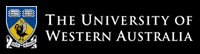School of Computer Science and Software Engineering

# School of Computer Science and Software Engineering

## CITS2200 Data Structures and Algorithms

### Some animals from the complexity zoo

ALL: the class of all languages

Almost-NP: the class of languages that is in NP with probability 1, given a random oracle

BPP: The class of problems for which there is a polynomial algorithm that is correct at least 2/3 of the time.

BQP: The class of problems solveable by a quantum Turing machine in polynomial time, correct at least 2/3 of the time.

Co-NP: The complement of NP

DSPACE(f(n)): The class of problems that may be solved by a Turing machine using O(f(n)) memory.

ELEMENTARY: Problems that can be computed in n-exponential time, for some n

EXP: Problems that can be computed in exponential time

FO: Problems that can be represented in first order logic

L: Problems that may be solved by a Turing machine memory logarithmic in the size of the problem.

NP: The class of dashed hopes and idle dreams (Languages that may be computed by a non-deterministic Turing machine in polynomial time)

P: Problems that may be solved using a deterministic Turing machine in polynomial time

RE: Problems that may be solved using a deterministic Turing machine in a finite amount of time

REG: Regular languages (languages that may be computed using a deterministic finite state machine)

WHILE: A theoretical programming in which all programmes are guarenteed to run in polynomial time.

ZPP: The class of problems that is expected to be solved in polynomial time by a randomized algorithm

1. Sahni, Chapter 10, Question 15

1. Write a singly-linked implementation of the deque ADT specified in Tutorial 3.

2. What is the complexity of each of the methods you have written?

3. What are the advantages and disadvantages of this approach over the cyclic block implementation of Tutorial 3?

2. Based on Sahni, Chapter 10, Questions 8 and 9

1. Write implementations of both the stack and queue ADTs by using the deque ADT.

3. Sahni, Chapter 10, Questions 8 and 9

1. Write implementations of both the stack and queue ADTs by sub-classing the deque ADT.